Copyright © Michael Richmond. This work is licensed under a Creative Commons License.

## Kinetic Energy and Work

• The kinetic energy of an object is defined as
```                           2
KE  =  1/2 * m * v
```
• The kinetic energy of an object depends on its velocity. To change its velocity, one must exert a force on it. It turns out there's a connection between the force one applies to an object and the resulting change in its kinetic energy:
```     KE(final) - KE(initial)  =  (force) (distance)
```
where the force is applied over some distance.
• We can this combination of force and distance work, so
```     KE(final) - KE(initial)  =  Work done on object
```
• In fact, it's a little more complicated than that. Only that component of the force which runs in the same direction as the displacement causes a change in the kinetic energy, so really
```     KE(final) - KE(initial)  =  (force parallel to displacement) (distance)
```
• Another way to write this is with vectors F for force and d for displacement. Then
```     KE(final) - KE(initial)  =   F  (dot product)  d

=   F * d * cos(alpha)
```
where alpha is the angle between vector F and vector d.

Viewgraphs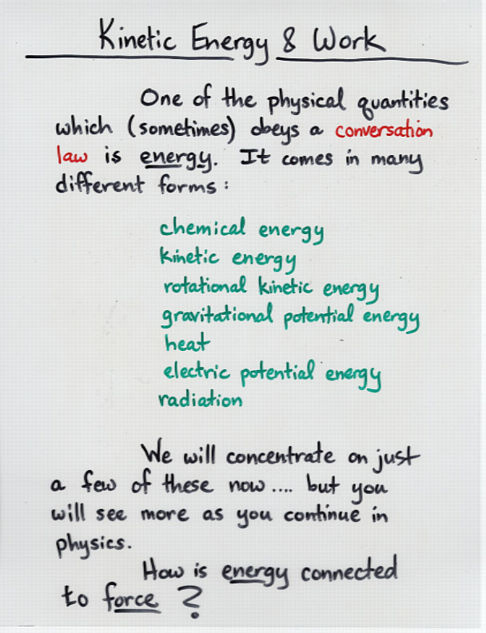Viewgraph 1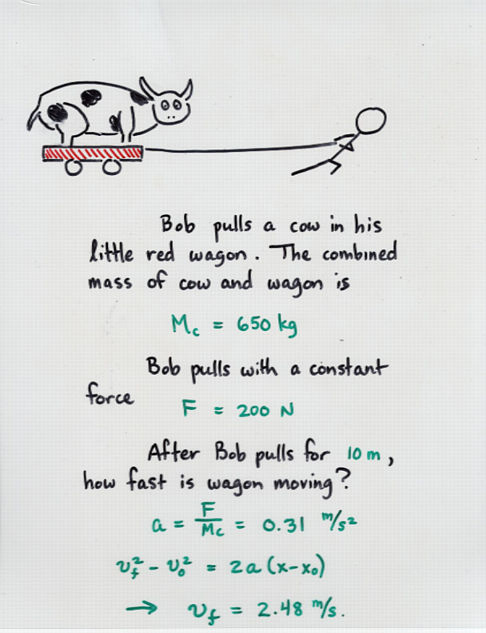Viewgraph 2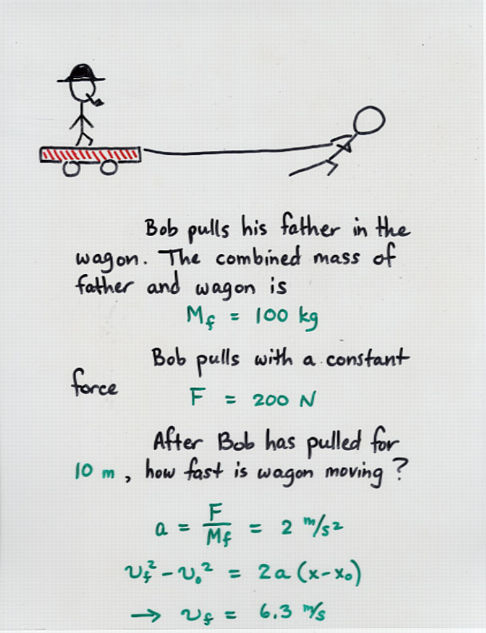Viewgraph 3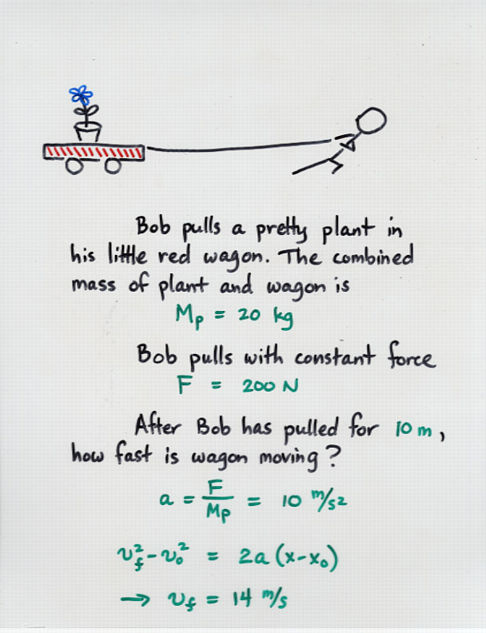Viewgraph 4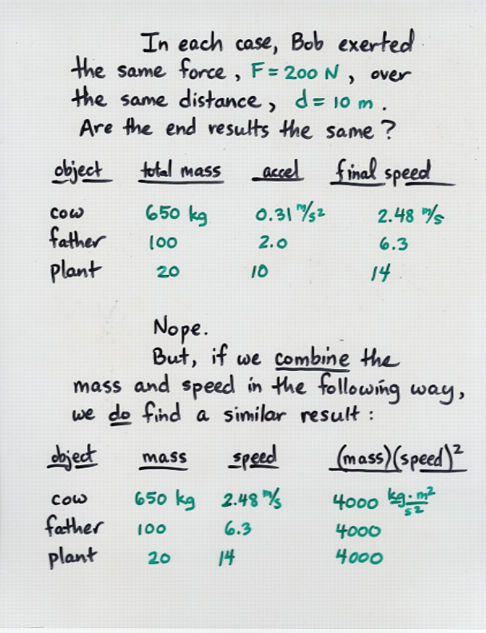Viewgraph 5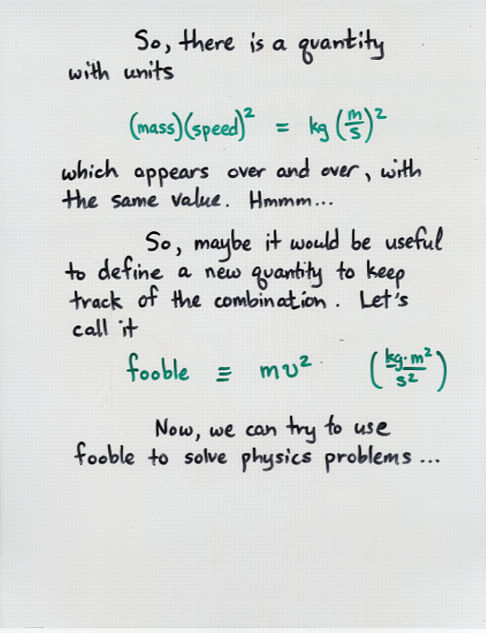Viewgraph 6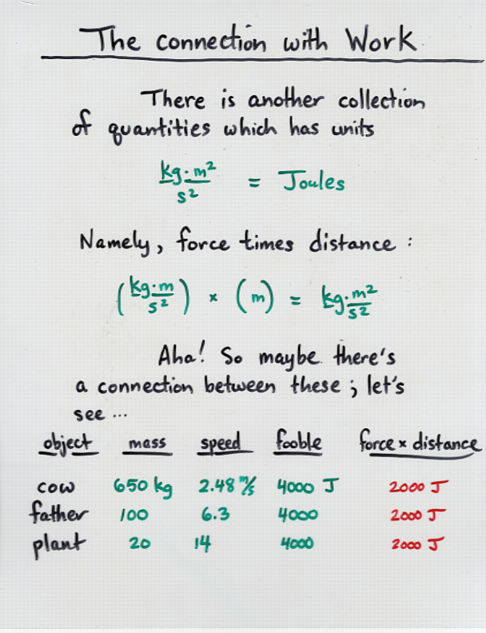Viewgraph 7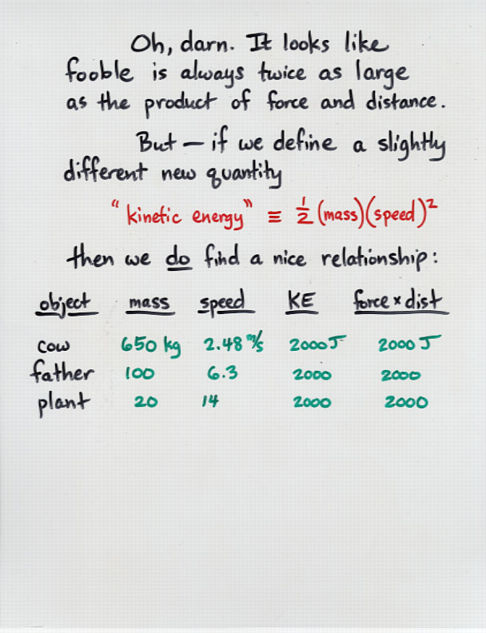Viewgraph 8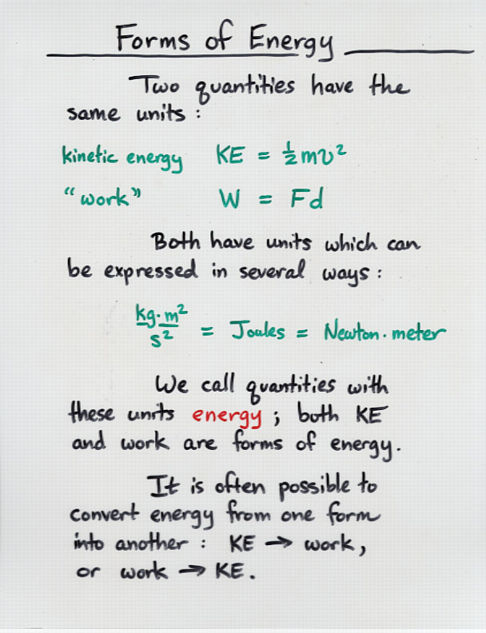Viewgraph 9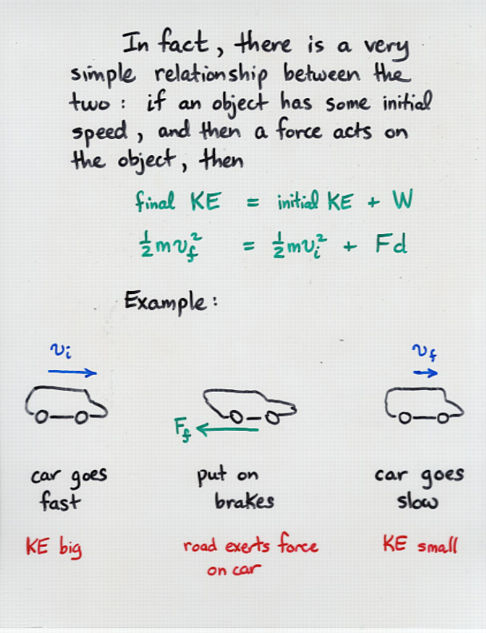Viewgraph 10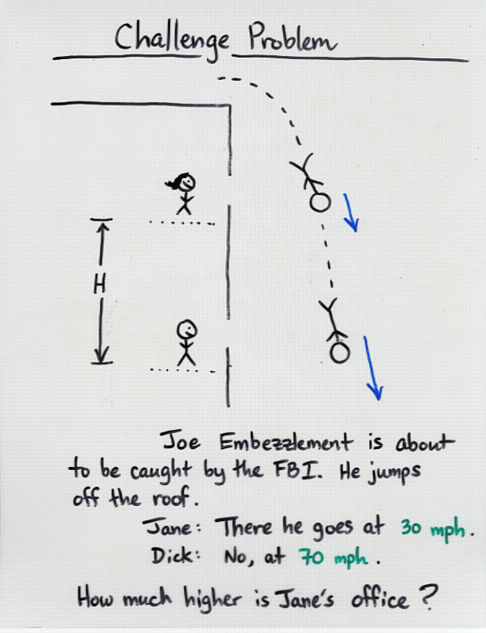Viewgraph 11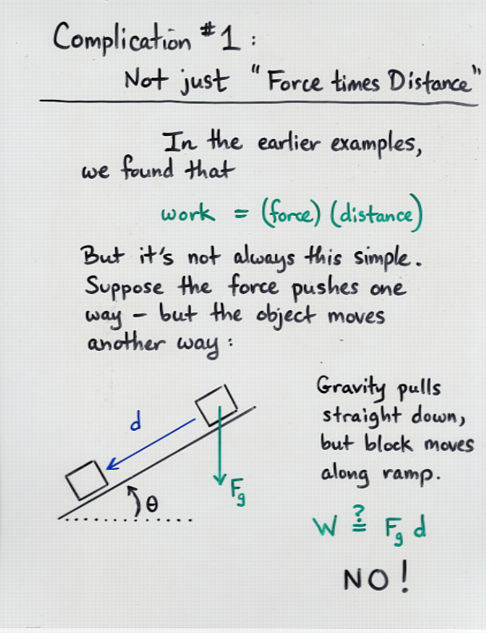Viewgraph 12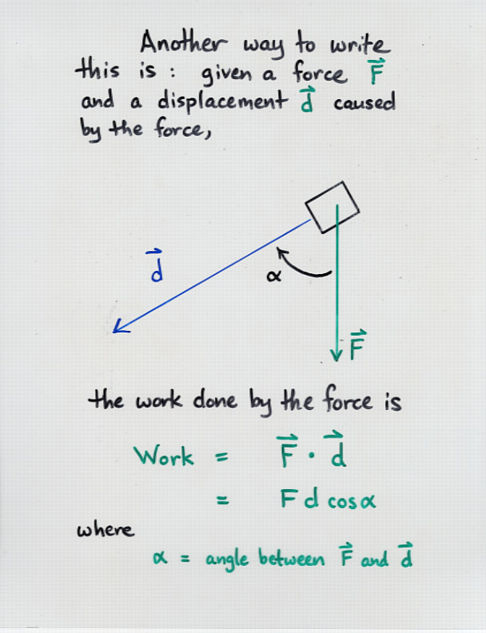Viewgraph 14Copyright © Michael Richmond. This work is licensed under a Creative Commons License.